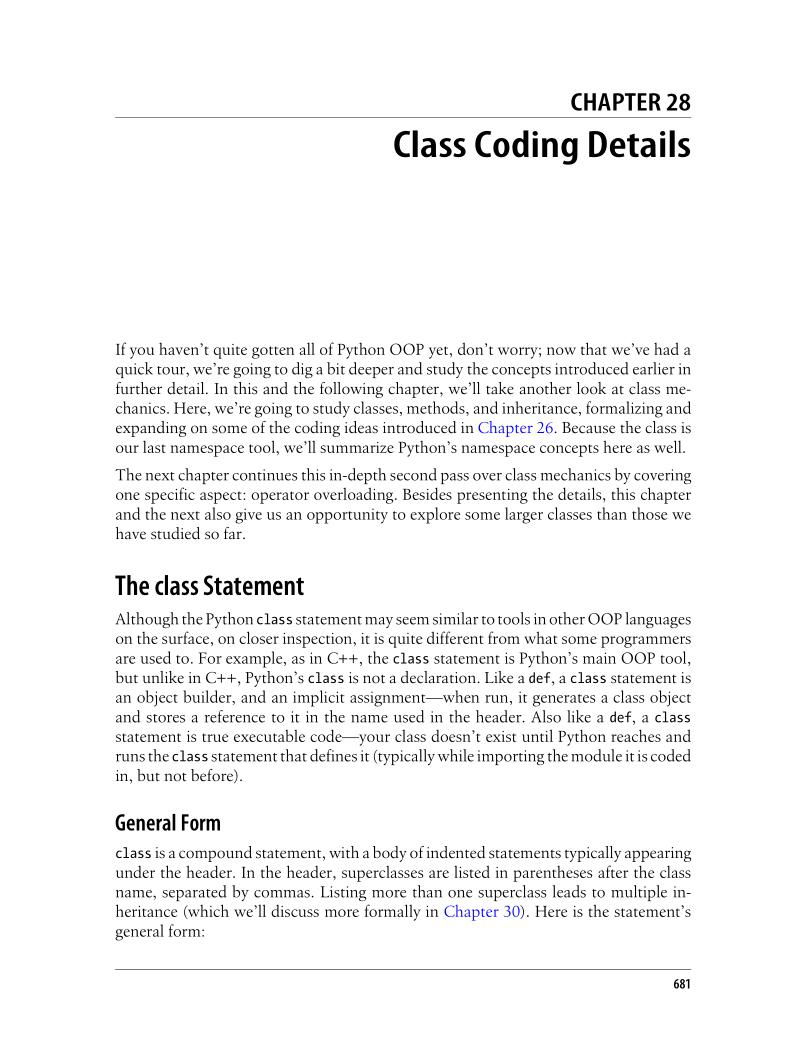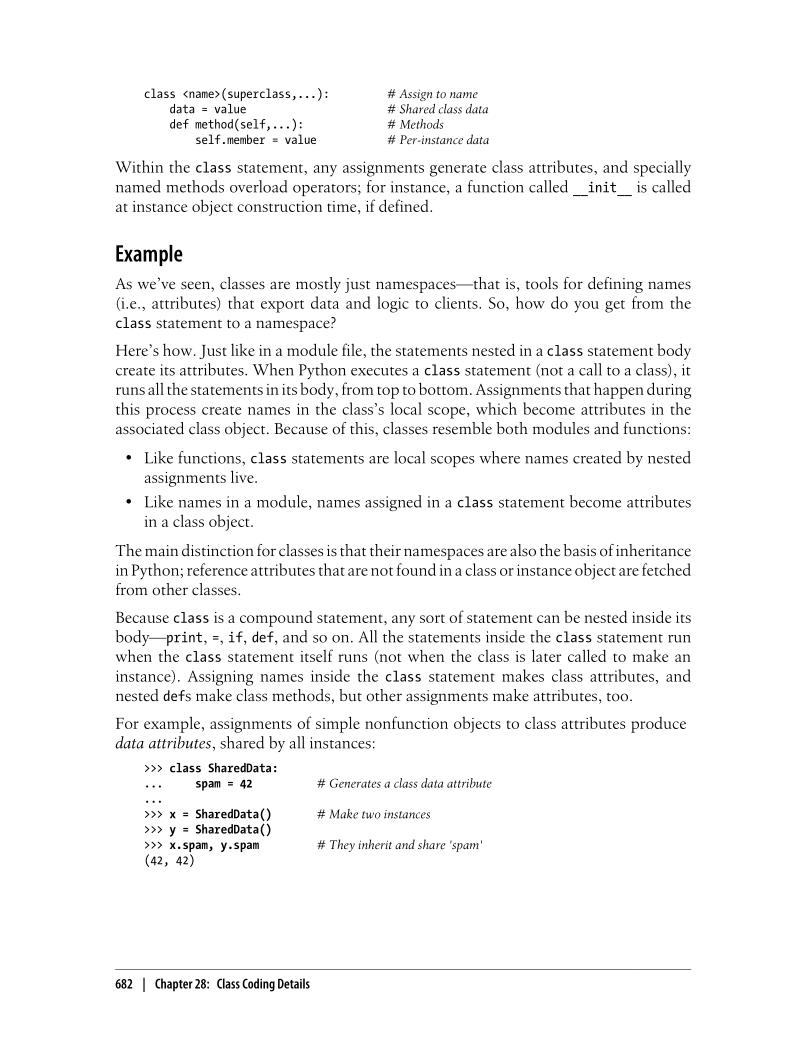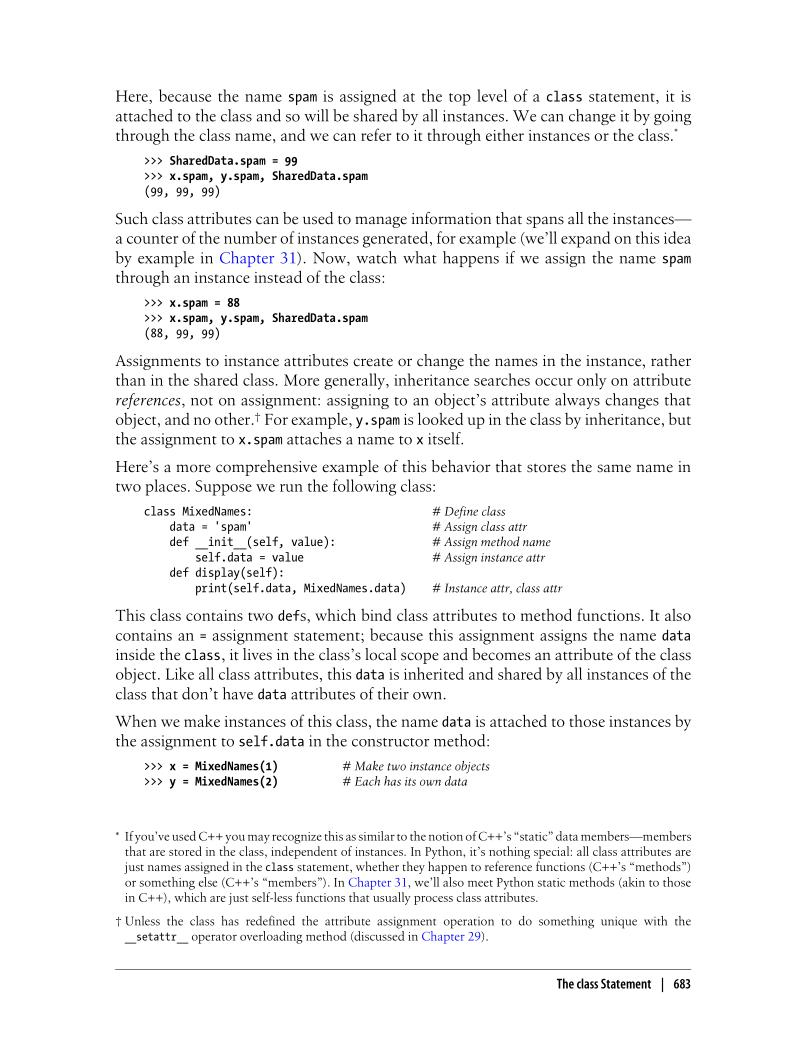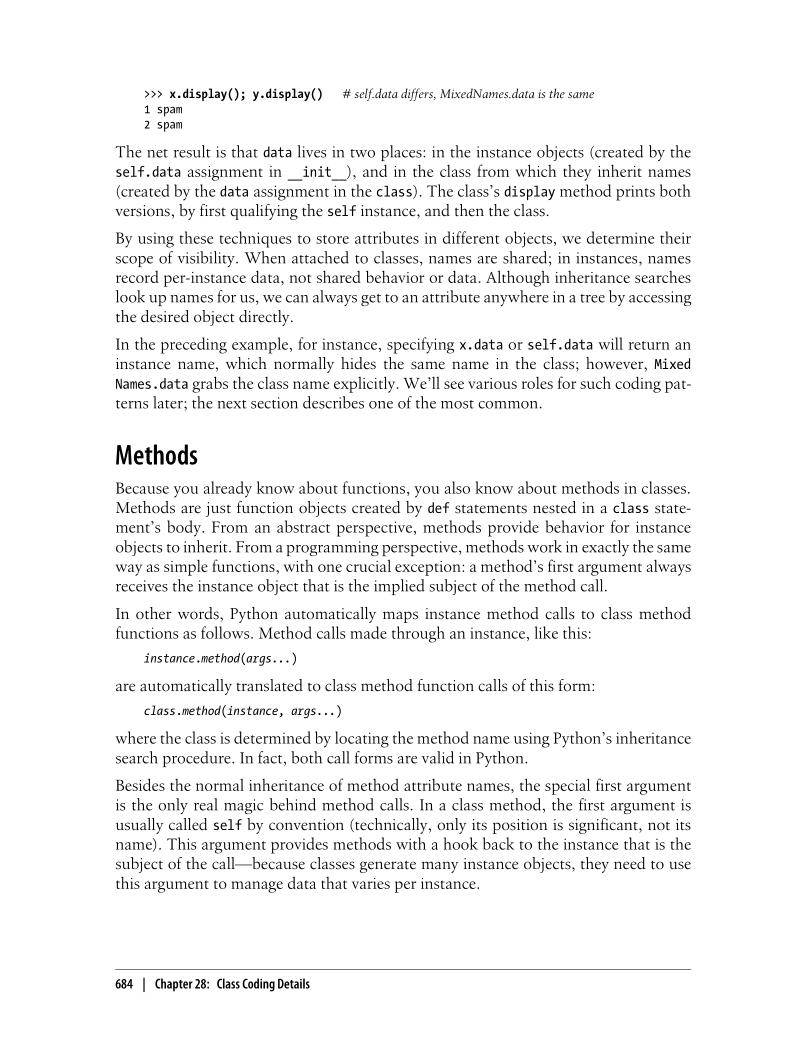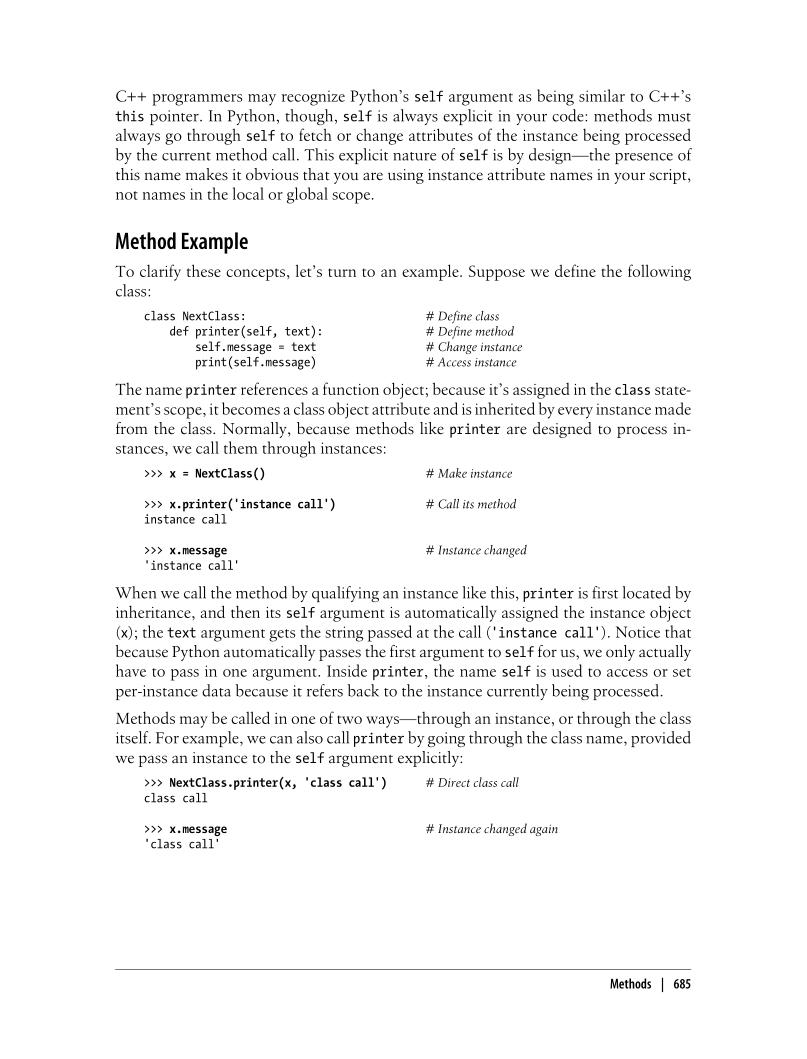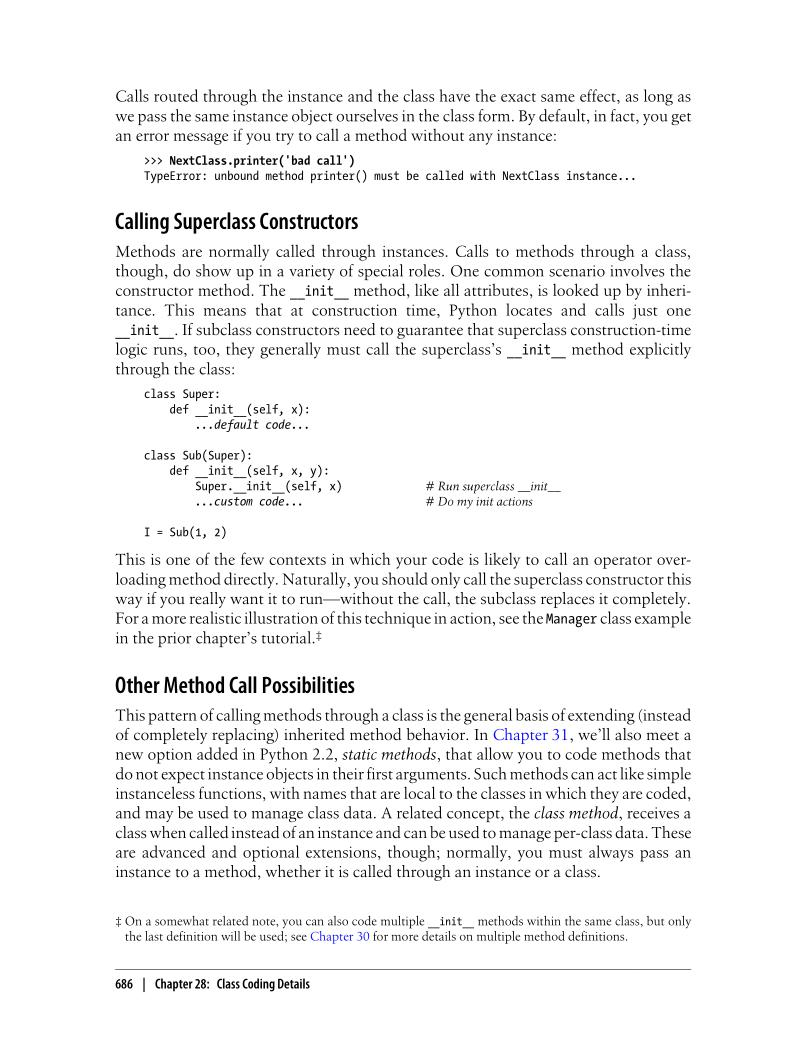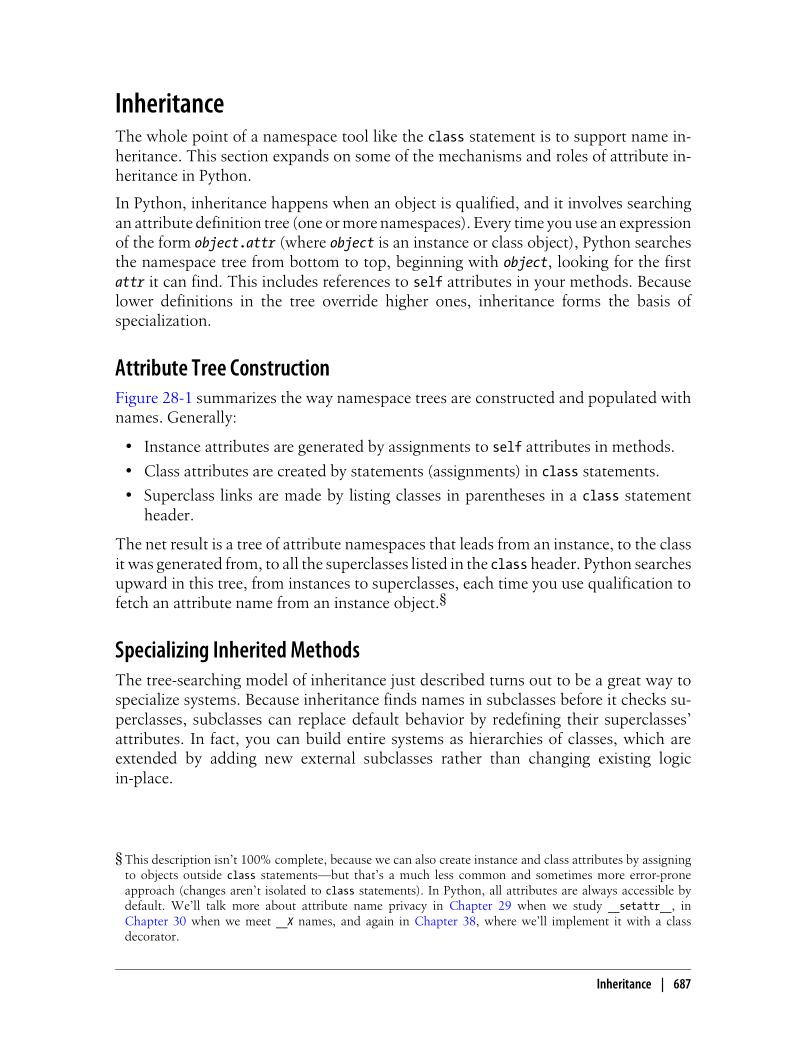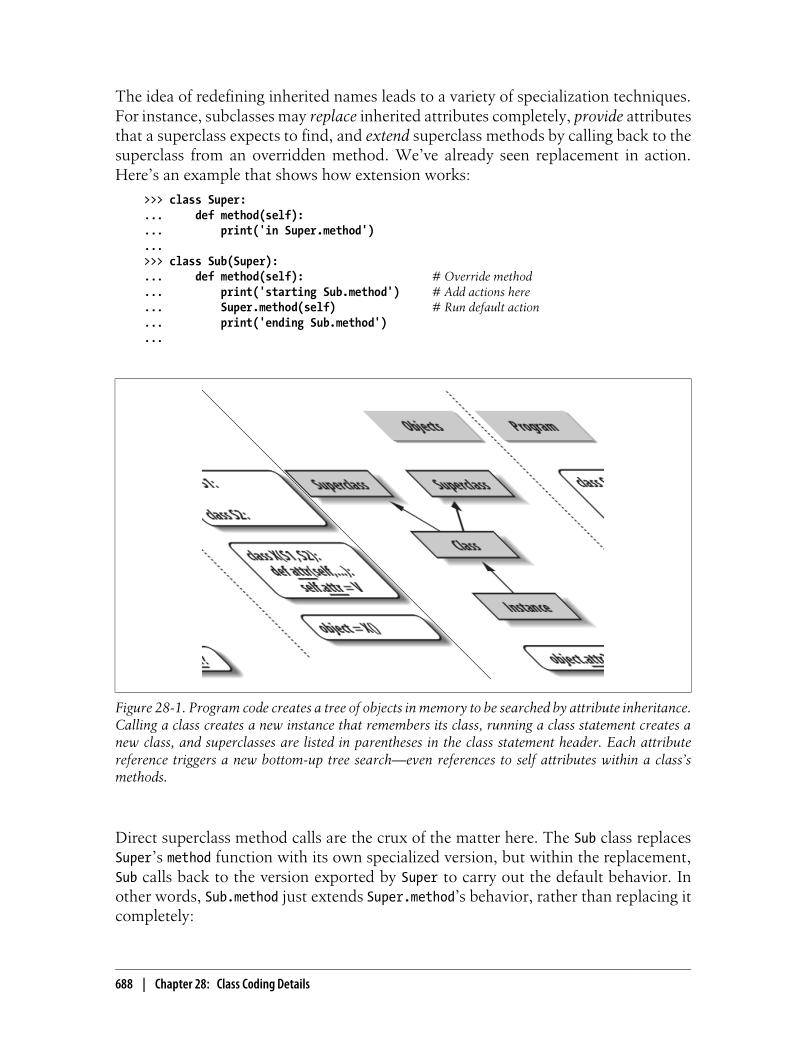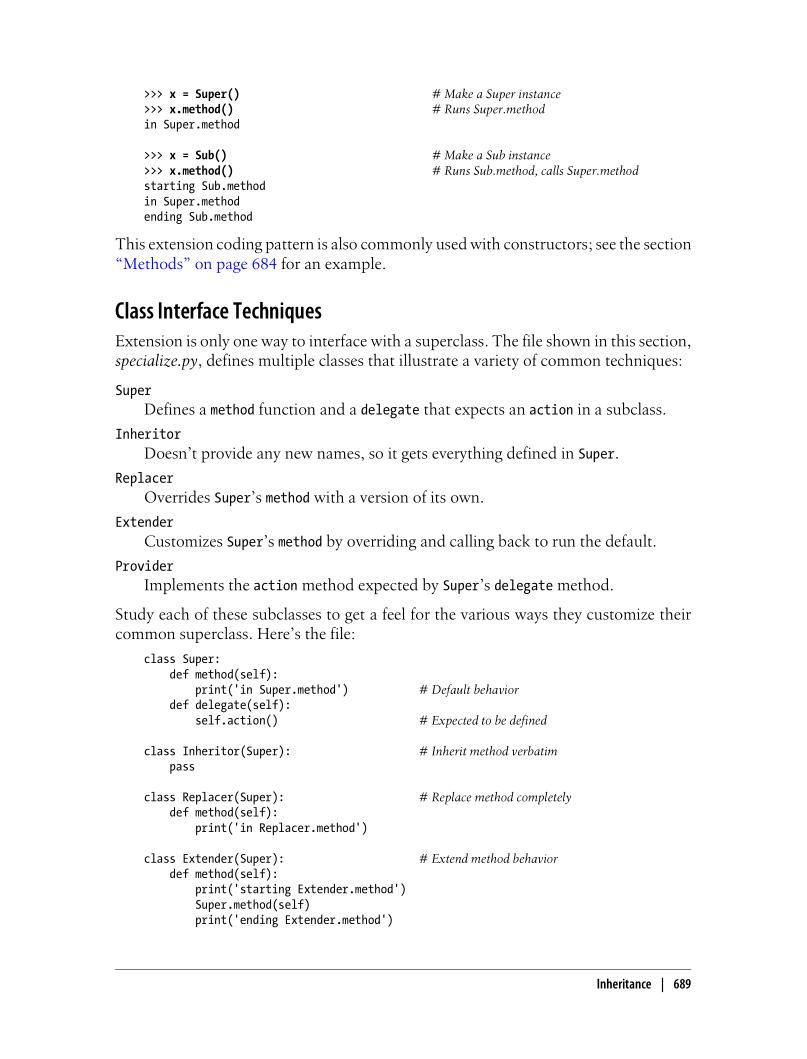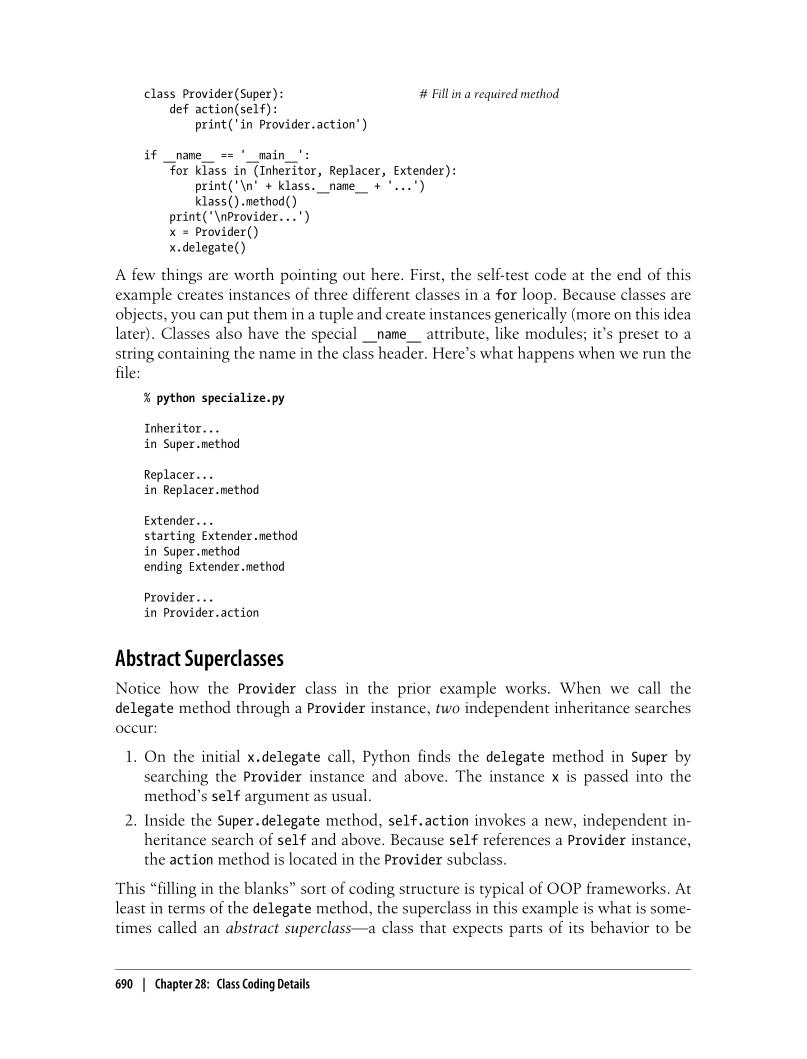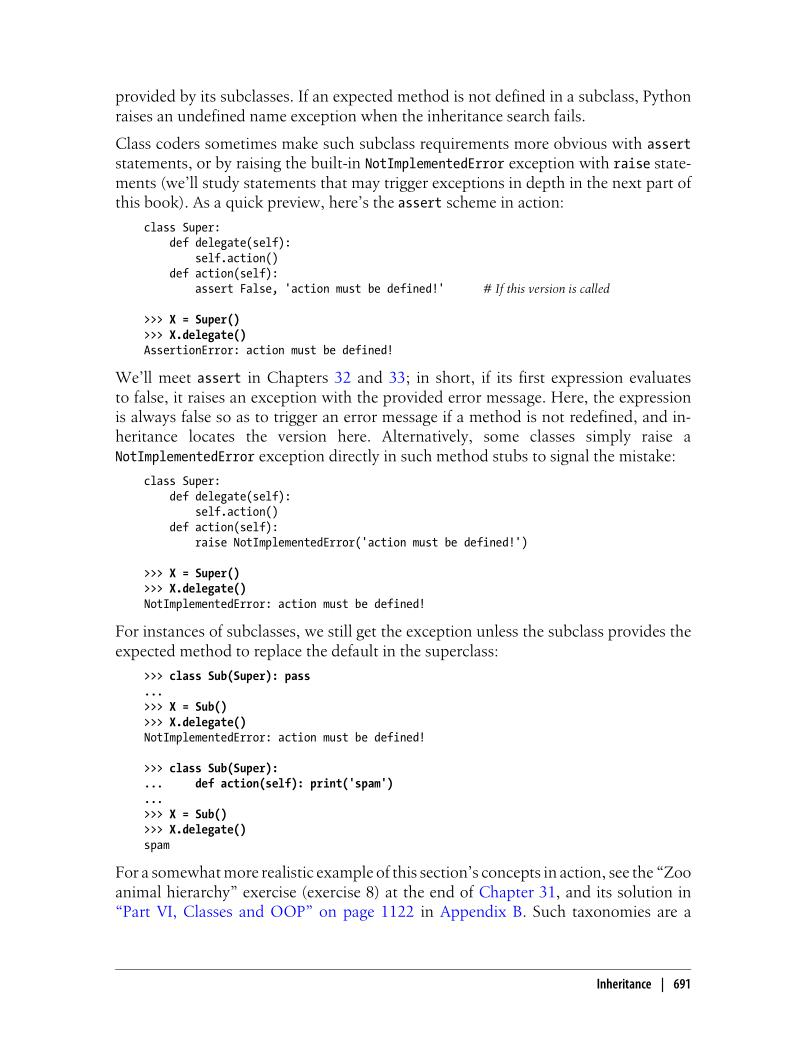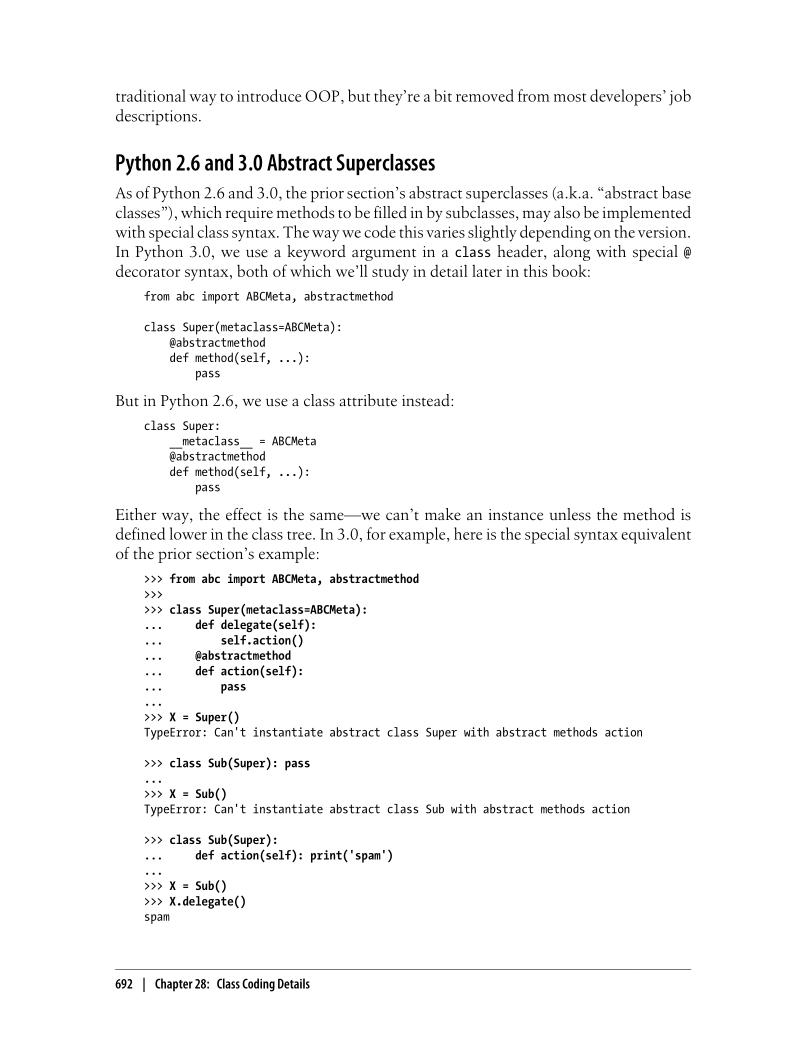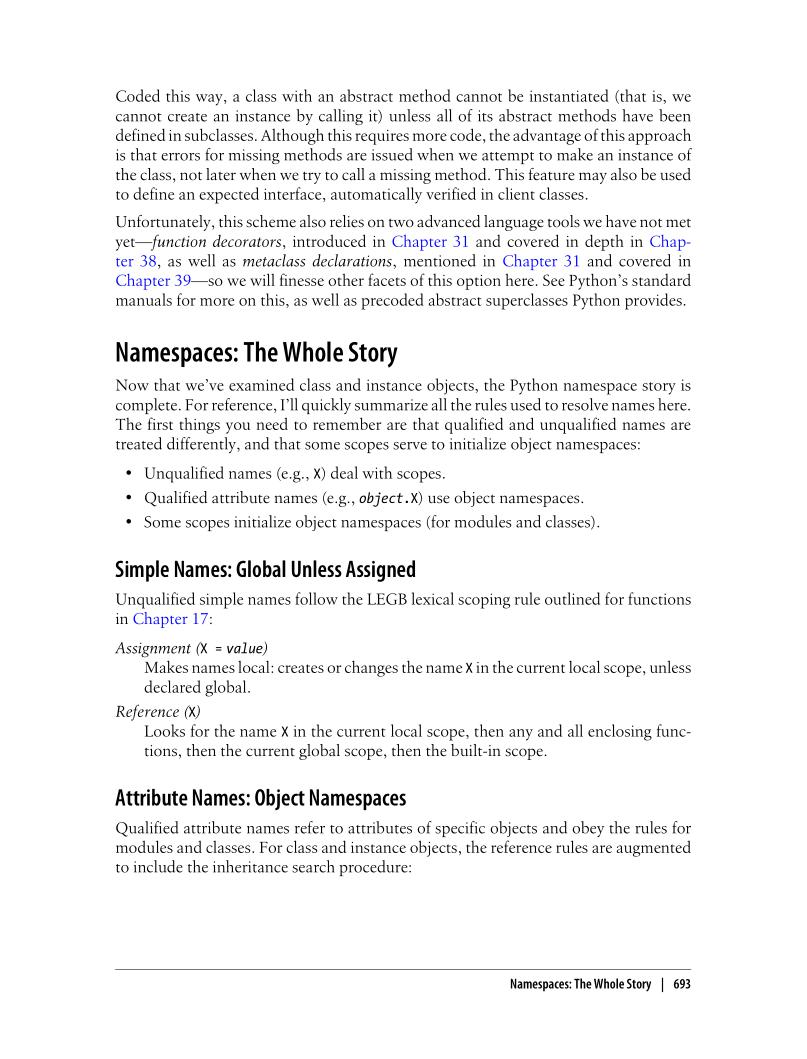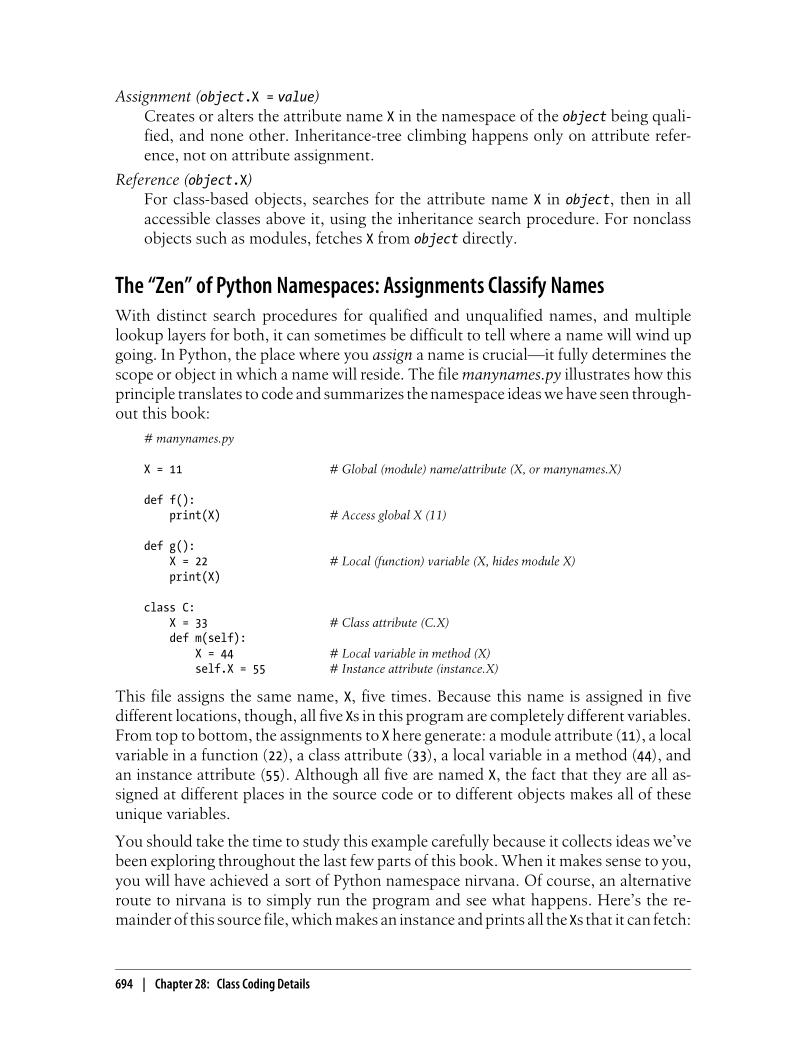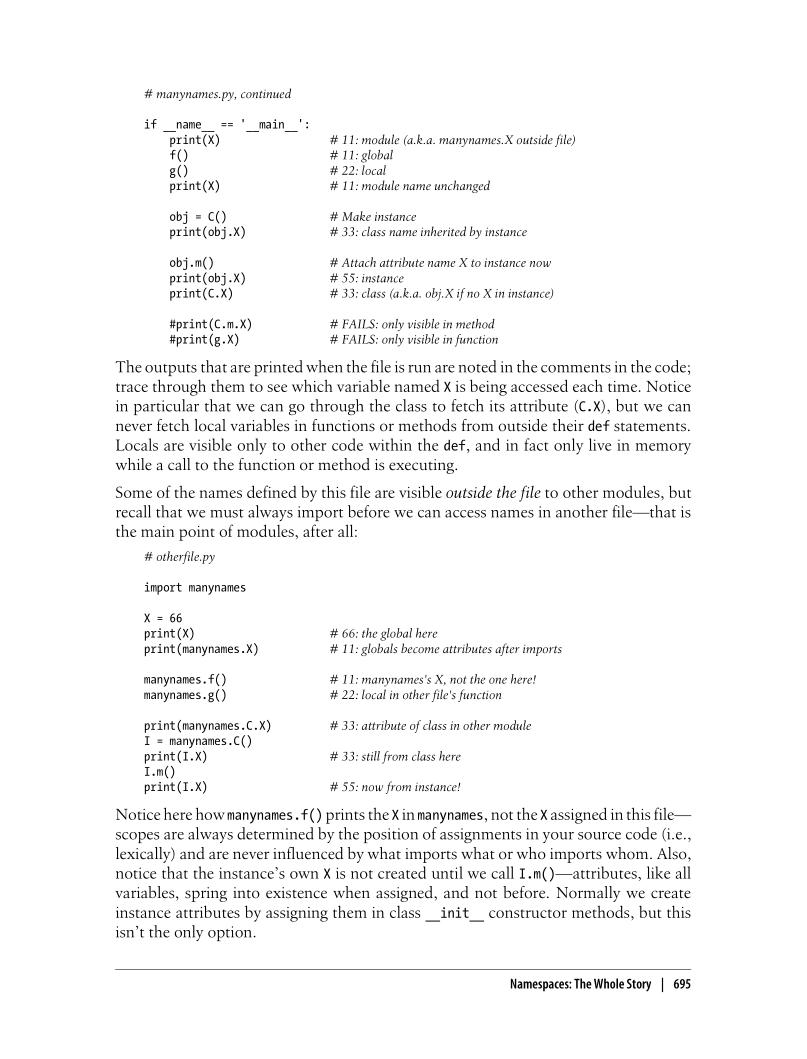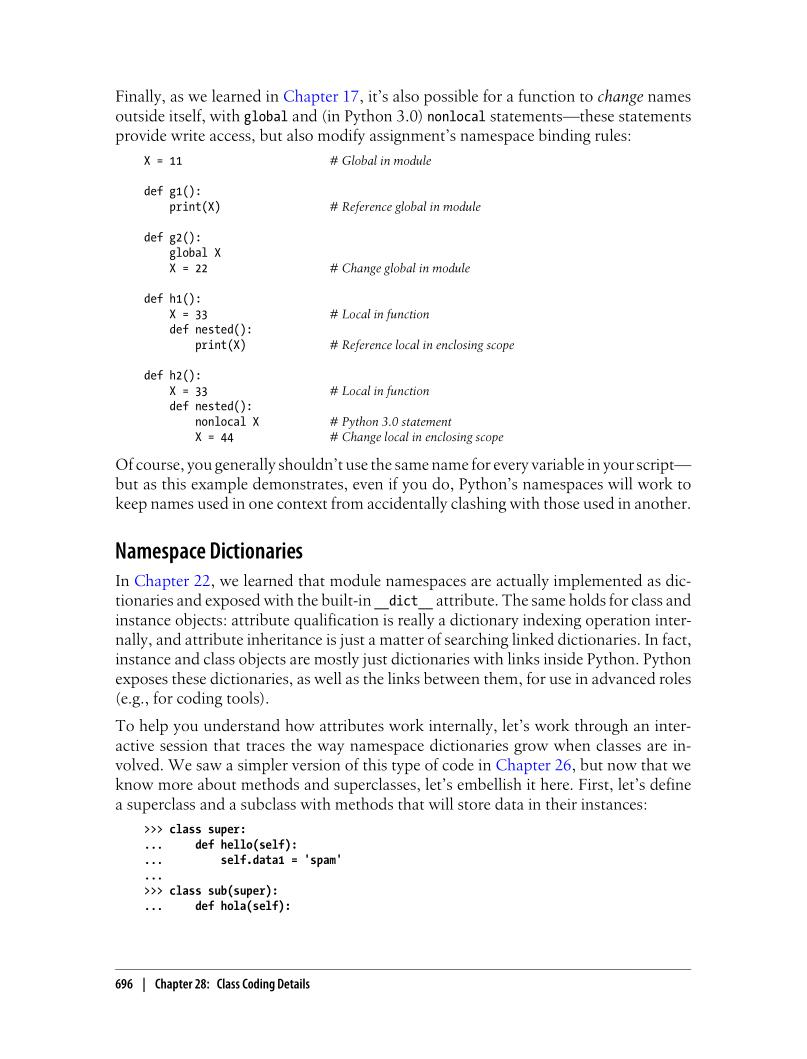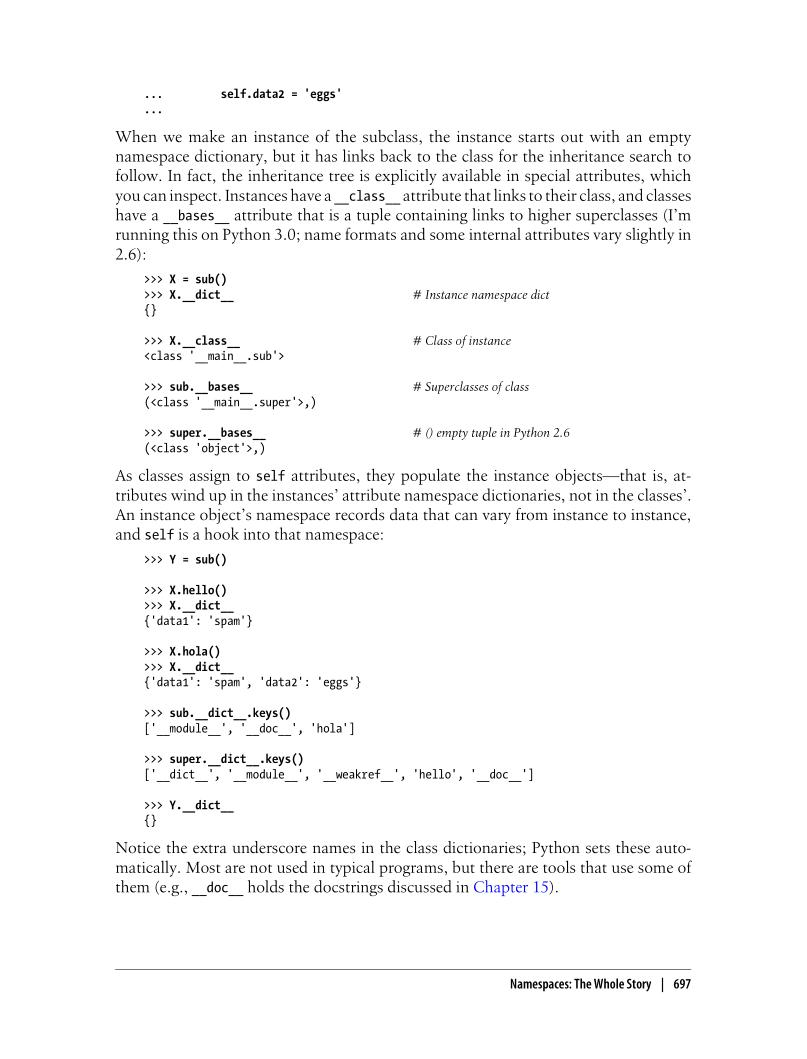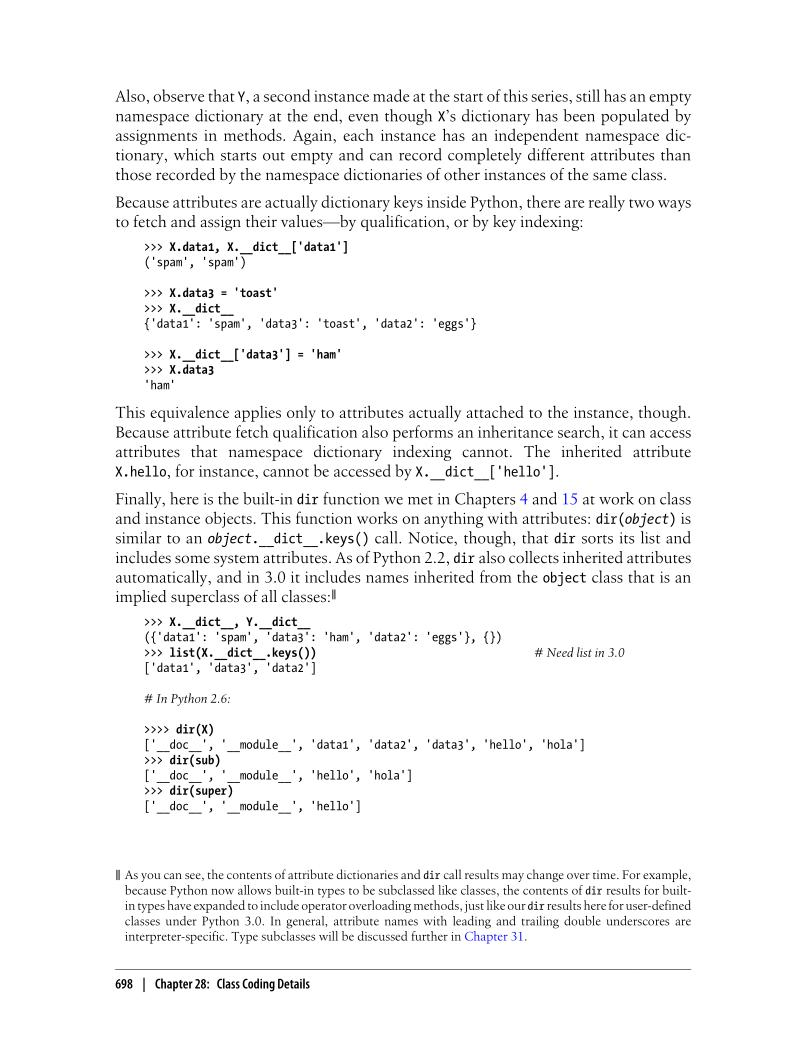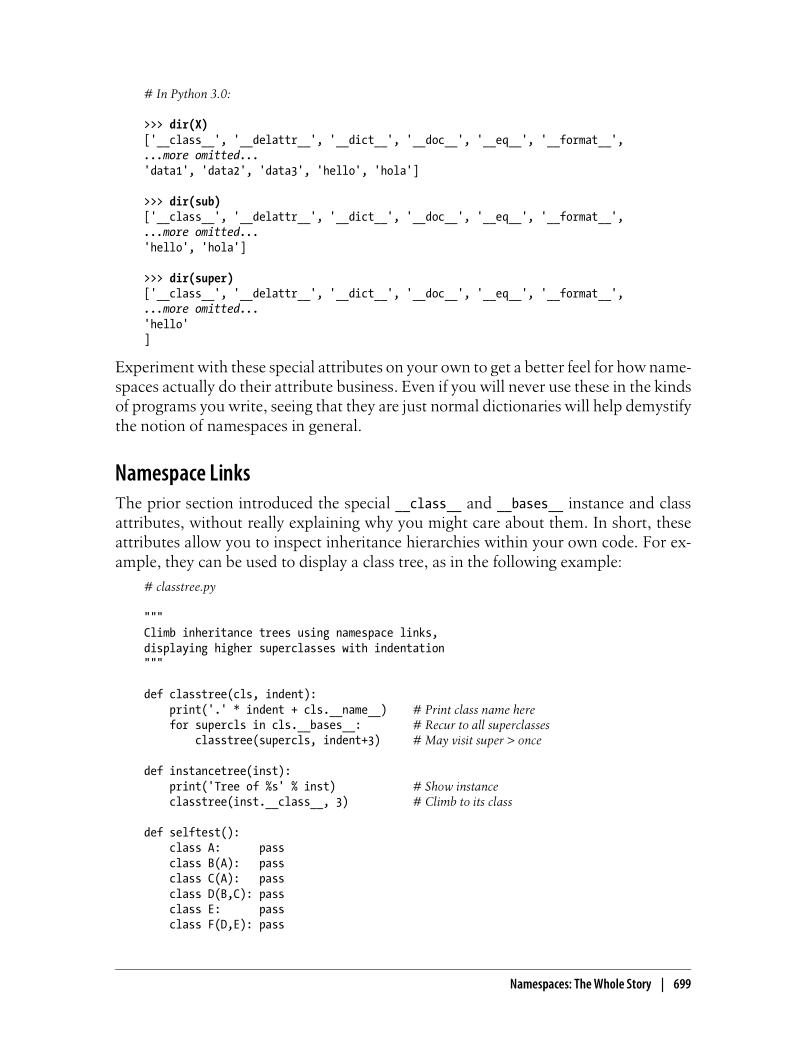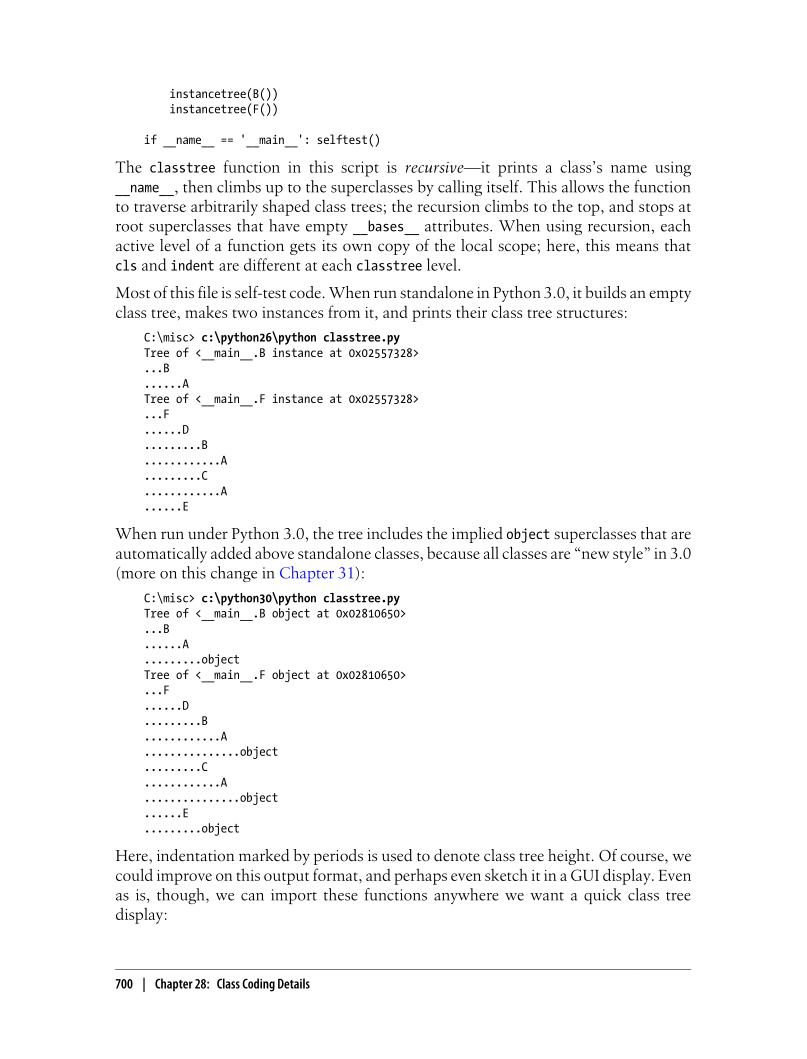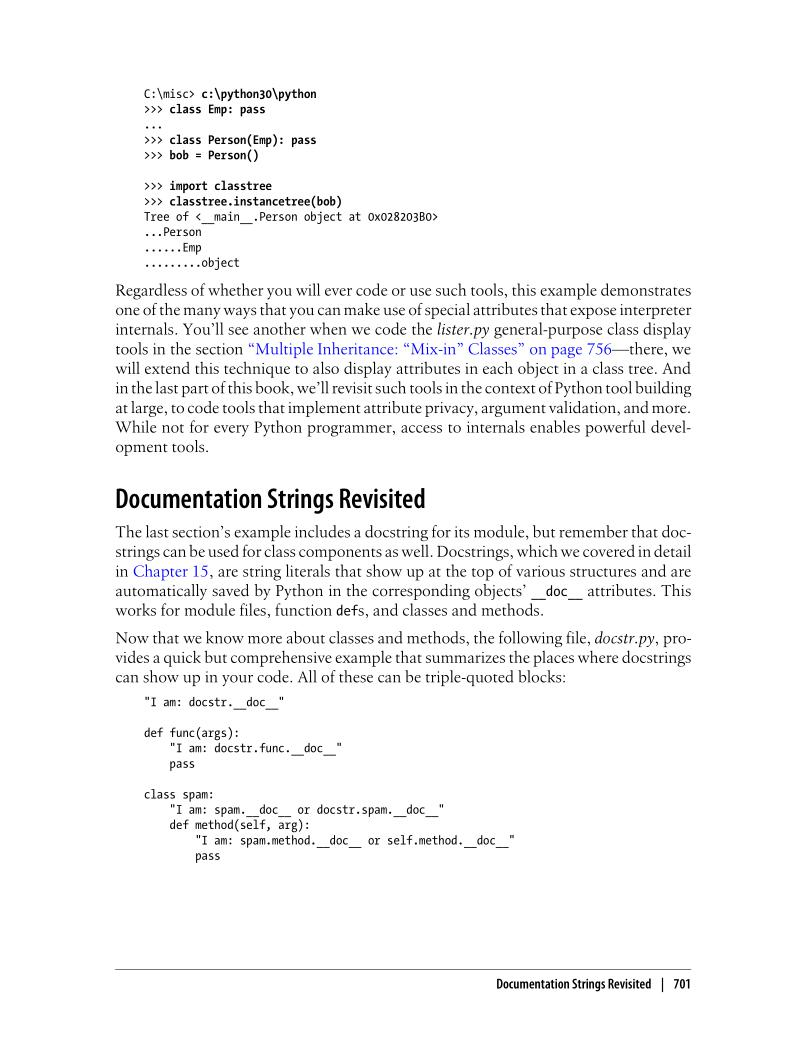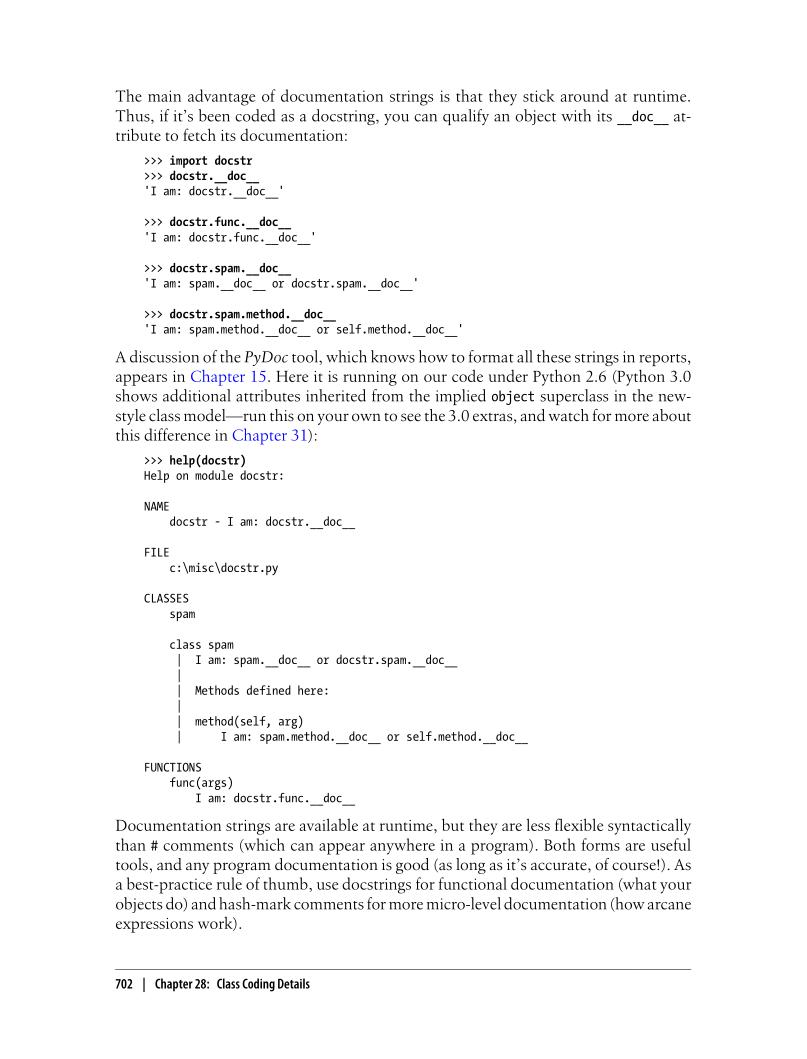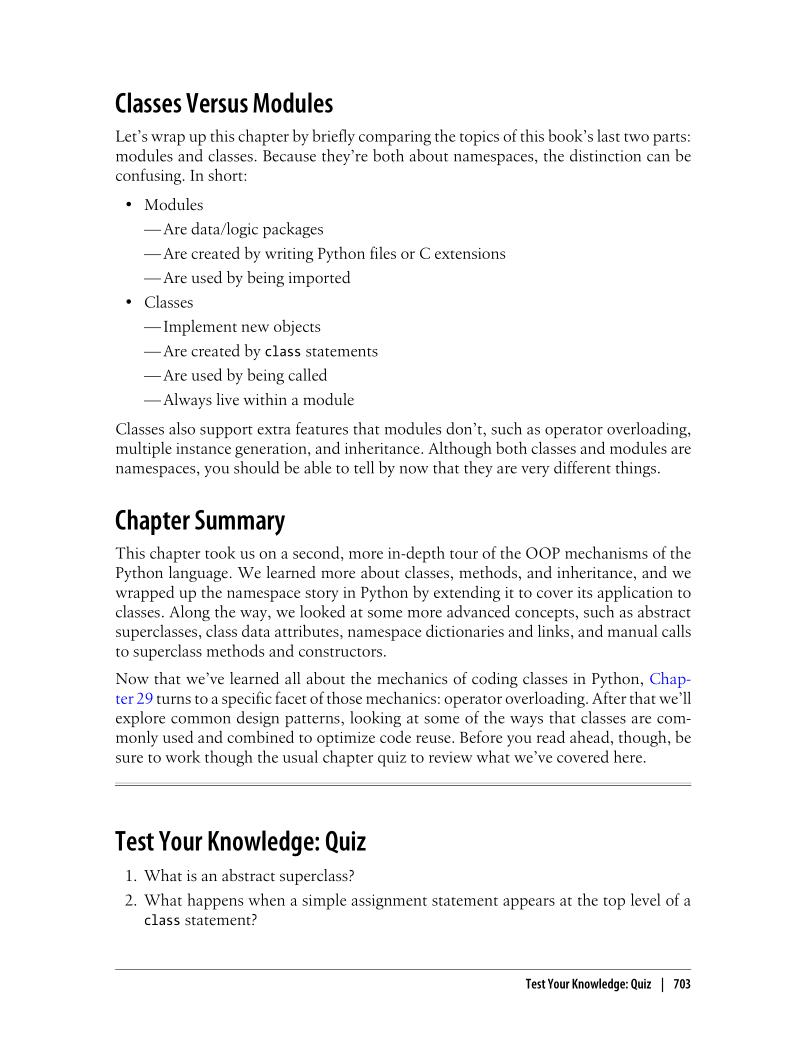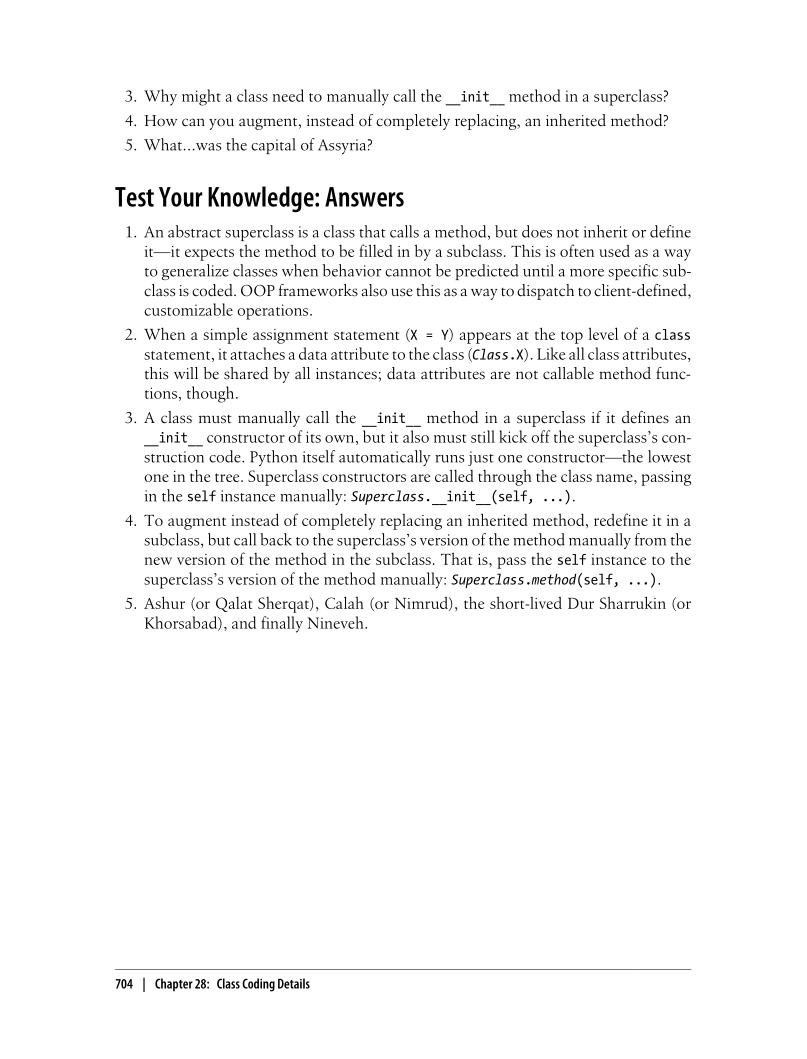# Chapter 28: Class Coding Details¶

As in C++. the class statement is Python’s main OOP tool, but unlike in C++, Python’s class is not a declaration. Like a def, a class statement is an object builder, and an implicit assignemnt-when run, it generates a class object and stores a reference to it in the name used in the header. when ru, your class exist.

The main distinction for classes is that their namespaces are also the basis of inheritance in a class or instance object are fetched from other classes.

All the statements inside the class statement run when the class statement itself runs (not when the class is later called to make an instance). Assigning names inside the class statement makes class attributes, and nested defs make class methods, but other assignments make attributes, too.

```>>> class sharedata:
data = 1
>>> sharedata.data
1
>>> class sharedata:
class bigdata:
data = 1
>>> sharedata.bigdata.data
1
```
```>>> class sharedata:
data = 78
>>> x = sharedata(); y = sharedata()
>>> x.data, y.data
(78, 78)
>>> x.data = 89; y.data = 91
>>> x.data, y.data, sharedata.data
```
```>>> classs <name> (superclass, ...):
data = value
def method(self,...):
self.member = value
```
```>>> class SharedData:
global spam
spam = 42
def __init__(self):
self.spam = spam
class subclass():
def __init__(self,spam):
super().__init__()
self.spam = spam
def __str__(self):
return str(self.spam)
>>> example1 = SharedData()
example1.spam
... 42
>>> example2 = example1.subclass(32)
>>> print(example2)
... 32
```
```# Methods
# Concept: methods provide behavior for instance objects to inherit
# Programming perspective: simple as functions, except that python automatically maps instance method calls to
# class method functions as follows.

>>> class NextClass:
def printer(self, text):
self.message = text
print(self.message)
>>> x = NextClass()
>>> x.printer('instance call')
... 'instance call'
>>> x.message
... 'instance call'

>>> dir(NextClass)
['__class__',
'__delattr__',
'__dict__',
'__dir__',
'__doc__',
'__eq__',
'__format__',
'__ge__',
'__getattribute__',
'__gt__',
'__hash__',
'__init__',
'__init_subclass__',
'__le__',
'__lt__',
'__module__',
'__ne__',
'__new__',
'__reduce__',
'__reduce_ex__',
'__repr__',
'__setattr__',
'__sizeof__',
'__str__',
'__subclasshook__',
'__weakref__',
'printer']
>>> NextClass.printer(x, 'class call') #Direct class call
>>> x.message
```